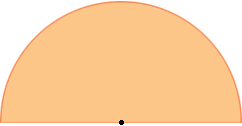SEARCH HOMEMath Central Quandaries & QueriesQuestion from sally, a student: Hi i am sally , i need to know what is the formula of : Find perimeter and area of a semicircle ? Find the area of a circle whose circumference ?Hi Sally,

The area of a circle of radius r is π r2 square units so the area of a semicircle of radius r is half of that. The perimeter of a circle of radius r, sometimes called the circumference of the circle, is 2 π r. The perimeter of a semicircle of radius r is composed of two pieces, curved piece and a straight piece. The length of the curved piece is half the circumference of the circle and the length of the straight piece is twice the radius.For your second question look at the reply I gave to Danny.

PennyMath Central is supported by the University of Regina and The Pacific Institute for the Mathematical Sciences.